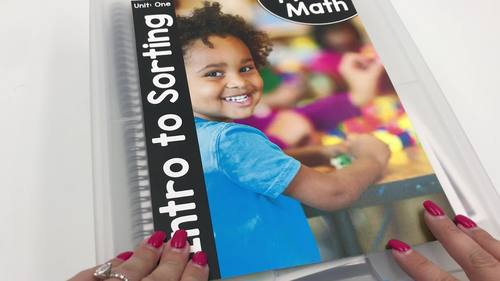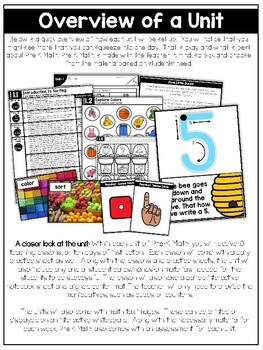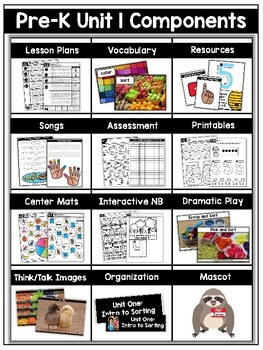Pre-K Math (Preschool Math) Curriculum UnitsSubject
Resource Type
Product Rating
File Type

Compressed Zip File

275 MB|2000+
Share
Product Description

Pre-K Math (Preschool Math) Curriculum Units- a preschool comprehensive math curriculum

HUGE \$100.00 SAVINGS FOR THE BUNDLE!

15 units @ \$12 each = \$180 - - SAVE \$100 BY PURCHASING THE BUNDLE!

What are fellow Pre-K teachers saying about Pre-K math?

"Words cannot express how AMAZING this resource is! Tara has thought of and INCLUDED EVERYTHING to make learning math fun and ENGAGING for our little learners from easy to read lesson plans, HANDS-ON DIFFERENTIATED small group plans, PLAY-BASED learning center ideas (using manipulatives many of us already have in our classrooms!), MUSIC and MOVEMENT, and adorable MASCOTS for each unit that the kids will absolutely love! There are so many things included that the only trouble will be figuring out how to fit it ALL in! It is a MUST HAVE resource for Pre-K and any Kindergarten or Sped teachers that like hands-on, minds-on learning! -Nicki"

UNIT ONE VIDEO

UNIT TWO VIDEO

UNIT FIVE VIDEO

UNIT SIX VIDEO

UNIT NINE VIDEO

UNIT TWELVE VIDEO

UNIT THIRTEEN VIDEO

UNIT FOURTEEN VIDEO

UNIT FIFTEEN VIDEO

What is Pre-K Math?

Pre-K Math units are teacher-created, kid-tested, and most importantly kid-approved! Pre-K Math takes away that monotonous way of teaching math where students sit and listen to the teacher TEACH math. Instead, Pre-K Math encourages guidance from the teacher while the students TEACH and LEARN math through hands-on and engaging math activities with their partners.

Pre-K Math units are made up of 10 instructional days each, but can be adjusted to fit your classroom needs. The units include assessment pieces as a way to ensure student growth over the unit.These are just a few highlights you’ll see using the Pre-K Math Units; kids talking about math, kids using manipulatives, activities that are fun + engaging, learning that requires critical thinking!

Pre-K Math curriculum is a 35 week math curriculum that includes lesson plans that break down a 60 minute block math time into these sections {movement, rhythm, whole-group lesson, small-group lesson and apply & practice}. The units will also include any and all cards needed to make the lessons successful! You will just need to provide the manipulatives like teddy bears or cubes!

Pre-K Math is a simple print and teach math curriculum. No more tracking down ideas, worksheets, cards, etc! I have it covered!

What is included in Pre-K Math?

- Teacher "simple read" lesson plans

- Each lesson I have planned out the instruction, song, differentiation and materials

- Assessment and assessment tracker

- Vocabulary cards

- Think and talk images

- Interactive notebook pages

- Aligned center mats

- Manipulative mats

- Daily printables

- Custom written INCLUDED books

Tell me more about the Pre-K Math Curriculum!

Lesson Plans: 10 sheets of lesson plans are included for each unit. You will also have access to editable lesson plans if you would like to edit.

Assessment and assessment tracker: A five question assessment is included. There is also a matching assessment tracker. The tracker can be used for informal or formal assessing.

Vocabulary cards: Each Pre-K Math unit includes a vocabulary focus. Vocabulary cards are included within the unit.

Think and talk images: Pre-K Math includes Think and Talk math images. These are built into the lessons.

Interactive notebook pages: Interactive notebooks pages are included for every math lesson.

Aligned center mats: LOW prep aligned center mats are included for every math lesson.

Dramatic play task cards: Dramatic task cards are included along with "I can" statements to promote hands-on learning throughout the math unit.

Manipulative mats: A variety of hands-on manipulative mats are included for each unit. The mats are open-ended and can utilize various manipulatives you already have access to.

Daily printables: Each lesson has an included practice sheet.

Custom INCLUDED books: Each unit has a custom included book. The book has a daily opportunity for students to apply the skill in a hands-on way and includes daily critical thinking starters.

How do the Pre-K Math lessons breakdown?

Movement: The students will be UP as the move and learn mathematical skills

Rhythm: Each week the students will be introduced to a new song

Whole-Group Lesson: This part of the math lesson will occur with the students down at the carpet. The whole-group lesson includes 2-3 routines led by the teacher.

Small-Group Lesson: This part of the math lesson will occur with the students grouped into levels. The teacher will follow the included differentiated lesson plans to meet each student at their level.

Apply and Practice: These are the activities the students will be working on independently or in small groups as their fellow classmates meet with the teacher.

Bundle Units:

Unit One: Introduction to Sorting INCLUDED

Unit Two: Introduction to Shapes INCLUDED

Unit Three: Introduction to Patterns INCLUDED

Unit Four: Introduction to Position Words INCLUDED

Unit Five: Introduction to Comparing Numbers INCLUDED

Unit Six: Introduction to Measurement INCLUDED

Unit Seven: Complex Sorting INCLUDED

Unit Nine: Complex Shapes INCLUDED

Unit Ten: Teen Numbers and Complex Comparing INCLUDED

Unit Eleven: Complex Measurement INCLUDED

Unit Twelve: Estimation and Data INCLUDED

Unit Thirteen: Combining Sets INCLUDED

Unit Fourteen: Subtracting Sets INCLUDED

Unit Fifteen: Kindergarten Prep INCLUDED

A closer look at the unit lessons!

Unit One: Introduction to Sorting

Lesson 1.1 Introduction to Sorting

Lesson 1.2 Explore Colors

Lesson 1.3 Explore Sorting by Color

Lesson 1.4 Sorting Objects that are the Same

Lesson 1.5 Sorting Objects that are Different

Lesson 1.6 The Number 1

Lesson 1.7 The Number 2

Lesson 1.8 The Number 3

Lesson 1.9 The Number 4

Lesson 1.10 The Number 5

Unit Two: Introduction to Shapes

Lesson 2.1 2D Shapes: Squares

Lesson 2.2 2D Shapes: Triangles

Lesson 2.3 2D Shapes: Circles

Lesson 2.4 2D Shapes: Rectangles

Lesson 2.5 2D Shapes: Ovals

Lesson 2.6 The Number 1 and Curvy/Straight Shapes

Lesson 2.7 The Number 2 and Shape Attributes

Lesson 2.8 The Number 3 and Shape Transformations

Lesson 2.9 The Number 4 and Counting Shape Sets to 5

Lesson 2.10 The Number 5 and Creating Shapes

Unit Three: Introduction to Patterns

Lesson 3.1 Colors and Sorting

Lesson 3.2 Pattern Introduction

Lesson 3.3 Movement Patterns

Lesson 3.4 Sound Patterns

Lesson 3.5 Numbers to 5

Lesson 3.6 Creating a Pattern

Lesson 3.7 Copy a Pattern

Lesson 3.8 Repeat a Pattern

Lesson 3.9 Finish and Expand a Pattern

Lesson 3.10 Review of Numbers to 5

Unit Four: Introduction to Positional Words

Lesson 4.1 Introduction to Position Words

Lesson 4.2 Paige the Pig's Positional Words

Lesson 4.3 Spot's Positional Words

Lesson 4.4 Harry's Positional Words

Lesson 4.5 Rosie's Positional Words

Lesson 4.6 The Number 6

Lesson 4.7 The Number 7

Lesson 4.8 The Number 8

Lesson 4.9 The Number 9

Lesson 4.10 The Number 10

Unit Five: Comparing to 10

Lesson 5.1 Understanding More

Lesson 5.2 Understanding Less

Lesson 5.3 Understanding More, Less, and Same

Lesson 5.4 Comparing Sets for More

Lesson 5.5 Comparing Sets for Less

Lesson 5.6 Comparing Sets for More, Less, Same

Lesson 5.7 Comparing More Sets for More, Less, Same

Lesson 5.8 Building Sets to Match Numbers

Lesson 5.9 Comparing Numbers

Lesson 5.10 Comparing More Numbers

Unit Six: Introduction to Measurement

Lesson 6.1 Measuring Sizes

Lesson 6.2 Comparing Sizes

Lesson 6.3 Ordering Sizes

Lesson 6.4 Measuring Length

Lesson 6.5 Comparing Length

Lesson 6.6 Ordering Length

Lesson 6.7 Measuring Weight

Lesson 6.8 Comparing Weight

Lesson 6.9 Ordering Weight

Lesson 6.10 Nonstandard Length

Unit Seven: Complex Sorting

Lesson 7.1 Sorting by Color

Lesson 7.2 Sorting by Size

Lesson 7.3 Sorting by Shape

Lesson 7.4 Sorting by Weight

Lesson 7.5 Sorting by Texture

Lesson 7.6 The Sorting Rule

Lesson 7.7 Name the Sorting Rule

Lesson 7.8 Create a Sorting Rule

Lesson 7.9 Sort by More Than One Attribute

Lesson 7.10 Sort by More Than One Attribute

Unit Eight: Complex Patterns

Lesson 8.1 Identifying Patterns

Lesson 8.2 Duplicating Patterns

Lesson 8.3 Transferring Patterns

Lesson 8.4 Extending Patterns

Lesson 8.5 Creating Patterns

Lesson 8.6 Identifying the Pattern Core

Lesson 8.7 ABC Patterns

Lesson 8.8 AAB Patterns

Lesson 8.9 ABB Patterns

Lesson 8.10 Identifying Missing Pieces in a Pattern

Unit Nine: Complex Shapes

Lesson 9.1 2D Shapes Review

Lesson 9.2 Solid Shapes Introduction

Lesson 9.3 3D Shapes: Cubes

Lesson 9.4 3D Shapes: Spheres

Lesson 9.5 3D Shapes: Cones

Lesson 9.6 3D Shapes: Cylinders

Lesson 9.7 3D Shapes Review

Lesson 9.8 Comparing 3D Shapes

Lesson 9.9 Construct and Build 3D Shapes

Lesson 9.10 Flat Shapes in 3D Shapes

Unit Ten: Complex Comparing

Lesson 10.1 The Number 11

Lesson 10.2 The Number 12

Lesson 10.3 The Number 13

Lesson 10.4 The Number 14

Lesson 10.5 The Number 15

Lesson 10.6 The Number 16

Lesson 10.7 The Number 17 and Comparing for More

Lesson 10.8 The Number 18 and Comparing for Less

Lesson 10.9 The Number 19 and Comparing for the Same

Lesson 10.10 The Number 20 and Comparing for More, Less, and the Same

Unit Eleven: Complex Measurement

Lesson 11.1 Measuring Height

Lesson 11.2 Comparing Height

Lesson 11.3 Ordering Height

Lesson 11.4 Measuring Capacity

Lesson 11.5 Comparing Capacity

Lesson 11.6 Ordering Capacity

Lesson 11.7 Nonstandard Measurement

Lesson 11.8 Nonstandard Measurement

Lesson 11.9 Measurement Tools

Lesson 11.10 Measurement Tools Part 2

Unit Twelve: Estimation and Data

Lesson 12.1 Sort by One Attribute

Lesson 12.2 Sort by More Than One Attribute

Lesson 12.3 Eye Color Graphing

Lesson 12.4 Color Graphing

Lesson 12.5 The Graphing Process

Lesson 12.6 Creating a Graph

Lesson 12.7 Analyzing Data

Lesson 12.8 Estimation Introduction

Lesson 12.9 Hand Estimation

Lesson 12.10 Grab, Guess, and Count

Unit Thirteen: Combining Sets

Lesson 13.1 Combining Sets to 5

Lesson 13.2 Combining Sets to 5

Lesson 13.3 Combining Sets to 5

Lesson 13.4 Combining Numbers to 5

Lesson 13.5 Combining Sets to 10

Lesson 13.6 Combining Sets to 10

Lesson 13.7 Combining Numbers to 10

Lesson 13.8 Addition Symbols: Plus and Equal Signs

Lesson 13.9 Plus 1

Unit Fourteen: Subtracting Sets

Lesson 14.1 Subtracting Sets to 5

Lesson 14.2 Subtracting Sets to 5

Lesson 14.3 Subtracting Sets to 5

Lesson 14.4 Subtracting Sets to 5

Lesson 14.5 Subtracting Sets to 10

Lesson 14.6 Subtracting Sets to 10

Lesson 14.7 Subtracting Sets to 10

Lesson 14.8 Subtraction Symbols: Minus and Equal Signs

Lesson 14.9 Minus 1

Lesson 14.10 Subtraction Within 10

Unit Fifteen: Kindergarten Prep

Lesson 15.1 Counting by 2's

Lesson 15.2 Counting by 5's

Lesson 15.3 Counting by 10's

Lesson 15.4 Start and Stop Counting

Lesson 15.5 Counting Sets to 50

Lesson 15.8 Subtraction Word Problems

Lesson 15.9 Subtraction Word Problems

Lesson 15.10 Subtraction and Addition Word Problems

"What I love about this new PreK Math Curriculum is that Tara really thought about each and every piece of this including research and collaboration with preschool teachers to determine what would be best. At the end of the day, I appreciate that it is DEVELOPMENTALLY APPROPRIATE, and PLAY BASED. I can also say, that the small group differentiation is a HUGE time saver for planning. Don't make the mistake of NOT purchasing this curriculum...it's bound to be a game changer for you!" -Brent

Please view the preview to see the sample lesson plans/materials, and more!

Questions?

Would you like to receive notifications for my newly released packets and upcoming sale? Be sure to FOLLOW ME here on TPT!

**Join my private Facebook Group to join fellow Pre-K, Kindergarten, First Grade and Second Grade teachers that use my curriculum! Collaborate, chat and share ideas with like-minded teachers! Join the private Facebook group H E R E!

Visit my blog Little Minds at Work

Total Pages
2000+
N/A
Teaching Duration
1 Year
Report this Resource\$80.00